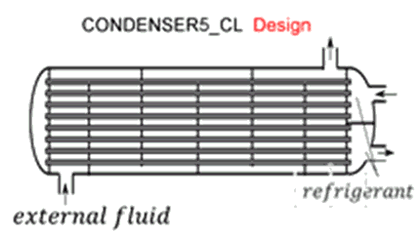Contents - index

Condenser5_CLCondenser5_CL determines the required heat transfer surface area to condense a superheated refrigerant to a specified degree of subcooling.  Thermal energy is rejected to an external fluid, which may be a liquid such as water or a brine.  It is necessary to provide the heat transfer coefficients on the external fluid side of the heat exchanger and for the superheated, saturated, and subcooled sections on the refrigerant side.  These heat transfer coefficients can be determined using the functions in the Heat Transfer Library, as illustrated in the example below.

This library file can be used in the design stage of the condenser by inputing the design inlet conditions to determine the required condenser size.  Use Condenser6_CL to determine the refrigerant and external fluid outlet states for given inlet conditions and condenser area.

Inputs:

R\$:   name of the refrigerant

m_dot_R:  refrigerant flow rate (kg/s, lbm/hr)

h_R_in:  specific enthalpy of entering refrigerant (J/kg, kJ/kg, Btu/lbm)

DELTAT_sc:  number of degrees of subcooling (C, K, F, R)

P_R:  refrigerant pressure (Pa, kPa, bar, MPa, psia, atm)

Config\$: a string constant or string variable that must be either 'counterflow' or 'parallelflow'

m_dot_fluid:  mass flow rate of the external fluid  (kg/s, lbm/hr)

T_fluid_in:  external fluid inlet temperature  (C, K, F, R)

cp_fluid:  specific heat of external fluid (J/kg-K, kJ/kg-K, Btu/lbm-R)

h_fluid:  effective outside (external fluid) heat transfer coefficient per unit refrigerant tube area (W/m^2-K, Btu/hr-ft^2-R)

A_fluid\A_R:  ratio of effective heat transfer area on the external fluid side to the heat transfer area for the refrigerant*

h_R_sh:  heat transfer coefficient for refrigerant in the superheated regime  (W/m^2-K, Btu/hr-ft^2-R)

h_R_sat:  average heat transfer coefficient for refrigerant in the saturated regime  (W/m^2-K, Btu/hr-ft^2-R)

h_R_sc:  average heat transfer coefficient for refrigerant in the subcooled regime  (W/m^2-K, Btu/hr-ft^2-R)

Outputs:

A_R:  required heat transfer area for the refrigerant (m^2, ft^2)

UA:  overall heat transfer coefficient (W/K ,Btu/hr-R)

Q_dot:  overall heat transfer rate (W, kW, Btu/hr)

h_R_out refrigerant outlet specific enthalpy (J/kg, kJ/kg, Btu/lbm)

T_fluid_out:  outlet temperature of the external fluid  (C, K, F, R)

f_sh:  fraction of the condenser area that is used for desuperheating

f_sc:  fraction of condenser area that is used for subcooling

*A_fluid\A_R is the ratio of the product of the overall fin efficiency and total (finned and unfinned) area on the external fluid side to the total surface area inside the refrigerant flow passages.  For unfinned surfaces, A_fluid\A_R is the ratio of the outer surface to inner surface areas.

Notes:

In actual operation, the heat exchanger type may shell-in-tube, plate or other type.  However, Config\$ may only be set to 'Parallelflow' or 'Counterflow'.  These two options provide upper and lower bounds on the required heat transer area.

Example:

\$unitSystem SI C kPa kJ mass

R\$='R134a'  "refrigerant"

P_R=1 [MPa]*convert(MPa,kPa)  "refrigerant pressure"

m_dot_R=0.075 [kg/s]  "refrigerant mass flow rate"

T_R_in=45 [C]  "inlet refrigerant temperature"

h_R_in=enthalpy(R\$,T=T_R_in,P=P_R)  "inlet refrigerant specific enthalpy"

DELTAT_sc=2 [C]  "subcooling at design"

Fluid\$='EG' "ethylene glycol-water is the external fluid"

Conc=20 [%] "concentration of the ethylene glycol - water solution"

T_fluid_in=20 [C]  "inlet temperature of external fluid"

Config\$='CounterFlow' "heat exchanger configuration"

m_dot_fluid=0.5 [kg/s] "mass flow rate of external fluid"

cp_fluid=cp(EG,T=T_fluid_in,C=Conc) "specific heat of external fluid"

h_fluid=620 [W/m^2-K]  "convection coefficient for external fluid side.  See h_fluid1 calculation below"

A_fluid\A_R=1.22 [-]  "ratio of area on external fluid side to refrigerant side.  See A_fluid\A_R1 calculation below"

h_R_sh=815 [W/m^2-K]  "convection coefficient for superheated R134a.  See h_T_sh calculation below"

h_R_sat=3825 [W/m^2-K]  "convection coefficient for saturated R134a.  See h_cond calculation below"

h_R_SC=970 [W/m^2-K]  "heat transfer coefficient in subcooled section.  See h_T_sc calculation below"

Call condenser5_cl(R\$, m_dot_R, h_R_in, DELTAT_sc, P_R, Config\$, m_dot_fluid, cp_fluid, T_fluid_in,  h_fluid, A_fluid\A_R, h_R_sh, h_R_sat, h_R_sc:  A_R, UA, Q_dot, h_R_out, T_fluid_out, f_sh, f_sc)

{!___________________________________________________________________________________________________}

"!Calculation of the heat transfer coefficients using the Heat Transfer Library functions"

N_circuits=4 "number of parallel circuits for refrigerant"

D_shell=0.175 [m] "diameter of shell"

W=0.3 [m]  "length of tubes"

N_tubes=round(A_R/(pi*W*D_in)) "required number of tubes"

D_o=1 [cm]*convert(cm,m) "outside tube diameter"

th = 0.9 [mm]*convert(mm,m)  "tube wall thickness"

D_in=D_o-2*th  "inner tube diameter"

A_fluid\A_R1=D_o/D_in "ratio of heat transfer area on external fluid side to that on refrigerant side"

"Determine heat transfer coefficients for the external fluid on the outside and refrigerant on the inside of the tubes"

T_R_sat=temperature(R\$,P=P_R,x=1) "saturation temperature of refrigerant"

Area_shell=pi*D_shell^2/4 "cross-sectional area of the heat exchanger shell"

Area_tubes=N_tubes*pi*D_o^2/4 "cross-sectional area of the refrigerant tubes"

Area_fluid=Area_shell-Area_tubes "free flow area for external fluid"

vel=m_dot_fluid/density(Fluid\$,T=T_fluid_in,C=Conc)/Area_fluid "velocity of external fluid"

"calculate heat transfer coefficient for the external fluid in a tube bank"

Pitch=D_o*1.2

G\$='SQUARE'

D_hp=4*(Area_shell-Area_tubes)/(pi*N_tubes*D_o+pi*D_shell)

Call tube_bundle(Fluid\$, T_fluid_in, Conc, G\$, Vel, D_o, Pitch, T_R_sat, W, D_hp:DELTAP_fluid, h_fluid1, Re, ,  )

"calculate the heat transfer coefficients for the refrigerant in the subcooled, saturated and superheat regimes"

m_dot_tube= m_dot_R/N_circuits "mass flow rate of refrigerant in one tube"

L_series=N_tubes*W/N_circuits "length of refrigerant flow path"

Call pipeflow(R\$,T_R_in, P_R, m_dot_tube, D_in, L_series*f_sh, 0: h_T_sh, , DELTAP_sh,  ,  , ) "superheat section"

Call cond_horizontaltube_avg(R\$, m_dot_tube, T_R_sat, T_fluid_in, D_in, 1, 0 : h_cond)           "saturated section"

Call pipeflow(R\$,T_R_sat-1 [C], P_R, m_dot_tube, D_in, L_series*f_sc, 0: h_T_sc,  , DELTAP_sc,  ,  , ) "subcooled section"

{!___________________________________________________________________________________________________}

{Solution

Area_fluid=0.01054

Area_shell=0.02405 [m^2]

Area_tubes=0.01351 [m^3]

A_fluid\A_R=1.22 [-]

A_fluid\A_R1=1.22

A_R=1.329 [m^2]

Conc=20 [%]

Config\$='counterflow'

cp_fluid=3.896 [kJ/kg-K]

DELTAP_fluid=0.01573

DELTAP_sc=0.05372

DELTAP_sh=1.949

DELTAT_sc=2 [C]

D_hp=0.007084

D_in=0.0082 [m]

D_o=0.01 [m]

D_shell=0.175 [m]

Fluid\$='EG'

f_sc=0.0216

f_sh=0.05849

G\$='SQUARE'

h_cond=3826 [W/m^2-K]

h_fluid=620 [W/m^2-K]

h_fluid1=618.1

h_R_in=277.3 [kJ/kg]

h_R_out=104.3 [kJ/kg]

h_R_sat=3825 [W/m^2-K]

h_R_SC=970 [W/m^2-K]

h_R_sh=815 [W/m^2-K]

h_T_sc=970.2

h_T_sh=816.5

L_series=12.9 [m^2]

m_dot_fluid=0.5 [kg/s]

m_dot_R=0.075 [kg/s]

m_dot_tube=0.01875 [kg/s]

N_circuits=4

N_tubes=172 [m]

Pitch=0.012 [m]

P_R=1000 [kPa]

Q_dot=12.97 [kW]

R\$='R134A'

Re=237.7

th=0.0009 [m]

T_fluid_in=20 [C]

T_fluid_out=26.66 [C]

T_R_in=45 [C]

T_R_sat=39.37 [C]

UA=815.00 [W/K]

vel=0.0463 [m^3/s]

W=0.3 [m]

}